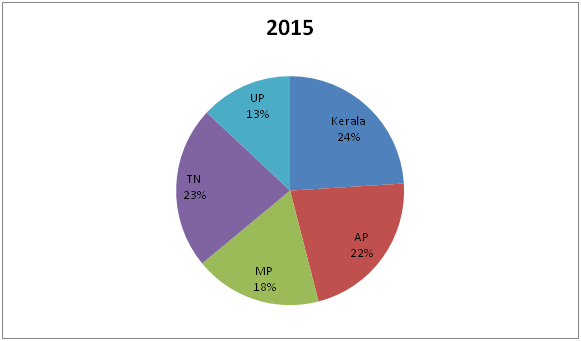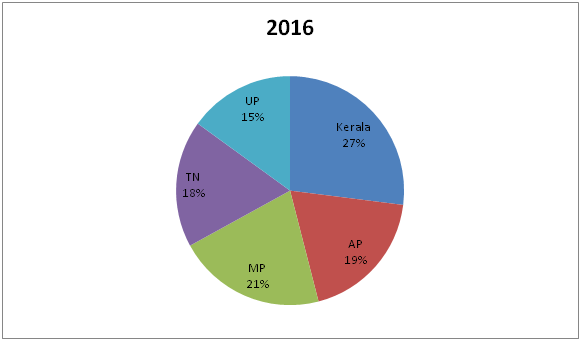# IBPS RRB Clerk/EPFO Assistant Prelims Quantitative Aptitude Questions 2019 (Day-08)

Dear Aspirants, Our IBPS Guide team is providing new series of Quantitative Aptitude Questions for IBPS RRB Clerk/EPFO Assistant Prelims 2019 so the aspirants can practice it on a daily basis. These questions are framed by our skilled experts after understanding your needs thoroughly. Aspirants can practice these new series questions daily to familiarize with the exact exam pattern and make your preparation effective.

##### EPFO Assistant Prelims Free Mock Test
[WpProQuiz 6683]

Directions (1 – 5): Find the approximate value in the place of question mark in the following questions.

1) 400.21.% of 184.91+34.75% of 240=?% of 1647.60

a) 30

b) 40

c) 82

d) 27

e) 50

2) (16.92)2+(59049)1/5-(429/13)=?

a) 246

b) 274

c) 346

d) 259

e) 265

3) (7.8/8.8)*(1.9/6.9)*(5.2/11.2)*38808/22=?

a) 1169

b) 1120

c) 1189

d) 1127

e) 1157

4) (5182)1/2-463.9÷7.7=2*?/7.9

a) 53

b) 92

c) 56

d) 39

e) 62

5) 40.25% of 2730+217.26% of 4200+(5832)1/3= (?)2+223.8

a) 100

b) 189

c) 178

d) 189

e) 167

Directions (6 – 10): Study the following information carefully and answer the given questions:

Following pie chart shows the distribution of total number of readers of a magazine in the year 2015 and 2016 among different states.6) If the total number of readers of the state AP in the year 2015 and 2016 is 57200 and 45600 respectively, then find the difference between the total number of readers of the state Kerala in the year 2015 to that of 2016?

a) 3200

b) 2700

c) 2400

d) 3500

e) None of these

7) If the ratio of total number of readers of the state AP in the year 2016 to that of the state MP in the year 2015 is 4 : 5, then find the ratio of total number of readers of all the given states together in the year 2016 to that in the year 2015?

a) 72: 95

b) 67: 88

c) 53: 92

d) 61: 76

e) None of these

8) If the total number of readers of all the given states together in the year 2015 and 2016 is 24 lakhs and 28 lakhs respectively, then find the total number of readers of the state MP in the year 2015 and 2016 together?

a) 11.5 lakhs

b) 14.6 lakhs

c) 17.3 lakhs

d) 10.2 lakhs

e) None of these

9) Total number of readers of state AP in the year 2016 is approximately what percentage of total number of readers of state MP in the year 2015?

(Assume that the total number of readers for both years is same.)

a) 94 %

b) 106 %

c) 88 %

d) 72 %

e) 118 %

10) If total number of readers of state Kerala in the year 2015 is 84000 and the total number of readers of state MP in the year 2016 is 52500, then the ratio of total number of readers of state AP in the year 2015 to that of total number of readers of state Kerala in the year 2016?

a) 57: 43

b) 81: 58

c) 65: 34

d) 77: 65

e) None of these

Direction (1-5) :

740+84=?% of 1648

824=?% of 1648

?=50

289+9-33=?

?=265

(8/9)*(2/7)*(5/11)*9702=?

14*2*8*5=?

?=1120

72-58=2*?/8

7*8=?

?=56

40% of 2730+217% of 4200+ (5832)1/3= (?)2+224

1092+9114+18=(?)2+224

10224-224=(?)2

?=100

Direction (6-10) :

The total number of readers of the state AP in the year 2015 = 57200

(22/100)*total number of readers in the year 2015 = 57200

Total number of readers in the year 2015 = 57200*(100/22) = 260000

The total number of readers of the state AP in the year 2016 = 45600

(19/100)*total number of readers in the year 2016 = 45600

Total number of readers in the year 2016 = 45600*(100/19) = 240000

Total number of readers of the state Kerala in the year 2015

= > 260000*(24/100) = 62400

Total number of readers of the state Kerala in the year 2016

= > 240000*(27/100) = 64800

Required difference = 64800 – 62400 = 2400

The ratio of total number of readers of the state AP in the year 2016 to that of the state MP in the year 2015 = 4: 5(4x, 5x)

Total number of readers in the year 2016 = 4x*(100/19) = 400x/19

Total number of readers in the year 2015 = 5x*(100/18) = 250x/9

Required ratio = (400x/19): (250x/9) = 72: 95

The total number of readers of all the given states together in the year 2015 is 24 lakhs

The total number of readers of MP in the year 2015 = 24*(18/100) = 4.32 lakhs

The total number of readers of all the given states together in the year 2016 is 28 lakhs

The total number of readers of MP in the year 2016 = 28*(21/100) = 5.88 lakhs

Required sum = 4.32 + 5.88 = 10.2 lakhs

Required % = (19/18)*100 = 105.55 % = 106 %

Total number of readers of state Kerala in the year 2015 = 84000

Total number of readers of state AP in the year 2015

= > 84000*(100/24)*(22/100)

= > 77000

Total number of readers of state MP in the year 2016 = 52500

Total number of readers of state Kerala in the year 2016

= > 52500*(100/21)*(27/100)

= > 67500

Required ratio = 77000: 65000 = 77: 65